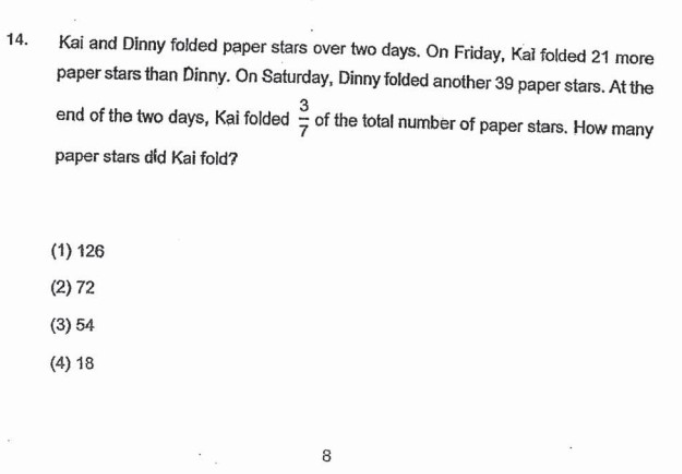# QuestionHow?

Happy New Year everybody!

In the above, we have simply assumed Kai folded 0 stars on Sat because he was not mentioned.  What if the question was meant to be a bit harder and we cannot assume that? The answer turns out to be the same. Read on if you are interested in this slightly harder variation. 🙂

Let the total number of stars folded be 7u. Then Kai folded 3u and Dinny folded 4u. That means Dinny folded 4u – 39 on Fri (because Fri + Sat = 4u) and Kai folded 4u – 18 (because he folded 21 more on Fri). So he must have folded 18 – u on Sat (in order for Fri + Sat to be 3u).

 Day Kai Dinny Fri 4u – 18 4u – 39 Sat 18 – u 39 TOTAL 3u 4u

Since all the number must be non-negative, we must have 18 – u >= 0, which means u <= 18. Also 4u – 39 >= 0, which means u >= 10. That means 10 <= u <= 18, implying 30 <= 3u <= 54. So of the 4 given options, only 54 is valid.

0 Replies 2 Likes ✔Accepted Answer

Kai                   Dinny

Friday              4U-39+21        4U-39

4U-18

Saturday         -1U+18            39

Total                3U                   4U

Given this is a multiple choice questions, we can solve by elimination of wrong answers.

18 is not possible as Kai had folded 21 more paper stars than Dinny on Friday, so 3U cannot be 18. So (4) is out.

U=126/3=42 is out also, as Kai folded 18-U on Saturday, Kai can never folded negative number.  so (1) out

U=72/3=24 is out also, so (2) is out  for same reason as previous.

So just left with (3) 54 as the answer, checking 54/3=18 meaning Kai folded 0 on Saturday and this answer is acceptable.

0 Replies 1 Like

This question did not specify how many stars Kai folded on Saturday. For simplicity, let’s assume he folded zero stars on Saturday.

Suppose Dinny folded x stars on Friday. Then Kai folded x + 21 on Friday. We draw up a table to record what we know:

 Day Kai Dinny Fri x + 21 x Sat 0 39 TOTAL x + 21 x + 39 (3 units) (4 units)

Kai folded 3/7 of the total number of stars. That means Dinny folded 4/7 of the total number of stars. If the total is 7 units, we can say that Kai folded 3 units and Dinny folded 4 units. In other words, Dinny folded 1 unit more that Kai.

From the table, we see that Dinny folded 18 more stars (difference between x + 39 and x + 21). So each unit is 18. Since Kai folded 3 units, he folded 3 x 18 = 54 stars altogether.